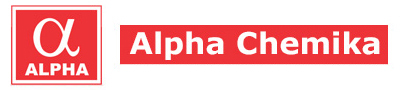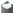: info@exporterlabchemicals.com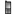: +91 98203 85757: +91 22 65218147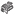: +91 22 26317055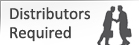0-9 A B C D E F G H I J K L M N O P Q R S T U V W X Y Z

Concentrated Volumetric Solutions

 » Edta Disodium Salt Dihydrate 0.1 mol/L » Hydrochloric Acid 0.1 mol/L (0.1N) for 500 ml solution » Hydrochloric Acid 1 mol/L (1N) for 500 ml solution » Iodine Solution 0.05 mol/L (0.1N) for 500ml solution » Oxalic Acid 0.05 mol/L (0.1N) for 500ml solution » Potassium Dichromate 0.0167 mol/L (0.1N) for 500ml solution » Potassium Permanganate 0.02 mol/L (0.1N) for 500ml solution » Silver Nitrate 0.1 mol/L (0.1N) for 500ml solution » Sodium Carbonate 0.05 mol/L (0.1N) for 500ml solution » Sodium Hydroxide 0.1 mol/L (0.1N) for 500ml solution » Sodium Hydroxide 1 mol/L (1N) for 500ml solution » Sodium Thiosulphate 0.1 mol/L (0.1N) for 500ml solution » Sulphuric Acid 0.5 mol/L (1 N) for 500ml solution » Sulphuric Acid 0.05 mol/L (0.1 N) for 500ml solution